We noticed that JavaScript is disabled in your browser. We suggest enabling it for a better experience.
We noticed you're using an older version of Internet Explorer. We suggest you update to the latest version for a better experience.

# Use calculations

Use calculations to figure out a value for something. Calculations can refer to other fields on your form. If you’re taking payments, they're the best choice for calculating how much to charge someone.

Add a calculation field to your form and give it a name, then we can go through how to use it.

## Calculation basics

Often, a calculation refers to other fields in the form and uses mathematical operators and functions to calculate a value based on those fields. You don't need to add fields to a calculation—you can just write a string of numbers, operators, and functions—but calculations work best with field information.

Here’s a basic calculation for calculating ticket cost:The calculation checks how many tickets the responder wants, then multiplies that number by ten, because one ticket costs \$10.

To build a calculation:

1. If you want, use the dropdown menu to add other fields from your form. Choose Number, calculation or date field to reference one of those field types; or choose Variable to reference a dropdown, checkbox or radio button field.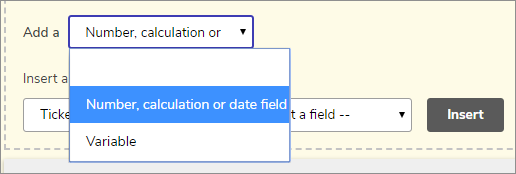2. If you’re adding a number, calculation or date field: Choose a section of your form, then a field within that section/group. Use Insert to add it to the calculation.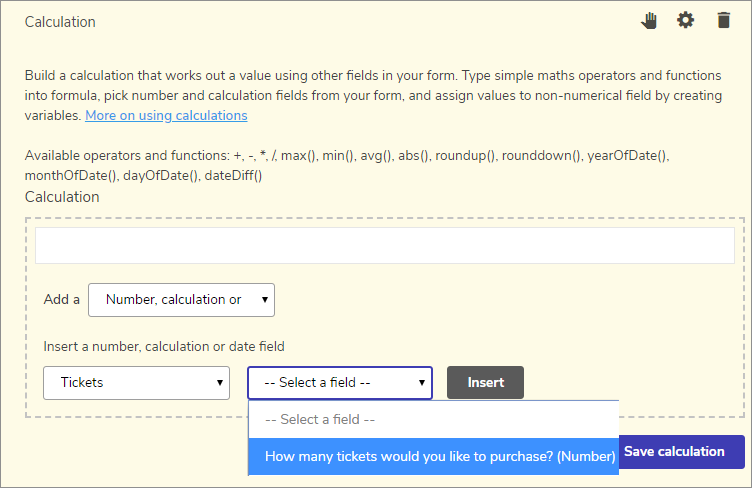If you're adding a value from a field within a repeatable group, it's worth noting that those work a little differently.

3. If you’re adding a variable: Choose a section of your form, then a field within that section. Use Create variable to assign values to each option, then use Create and insert to confirm it. For help with advanced values, jump to Make advanced calculations using variables.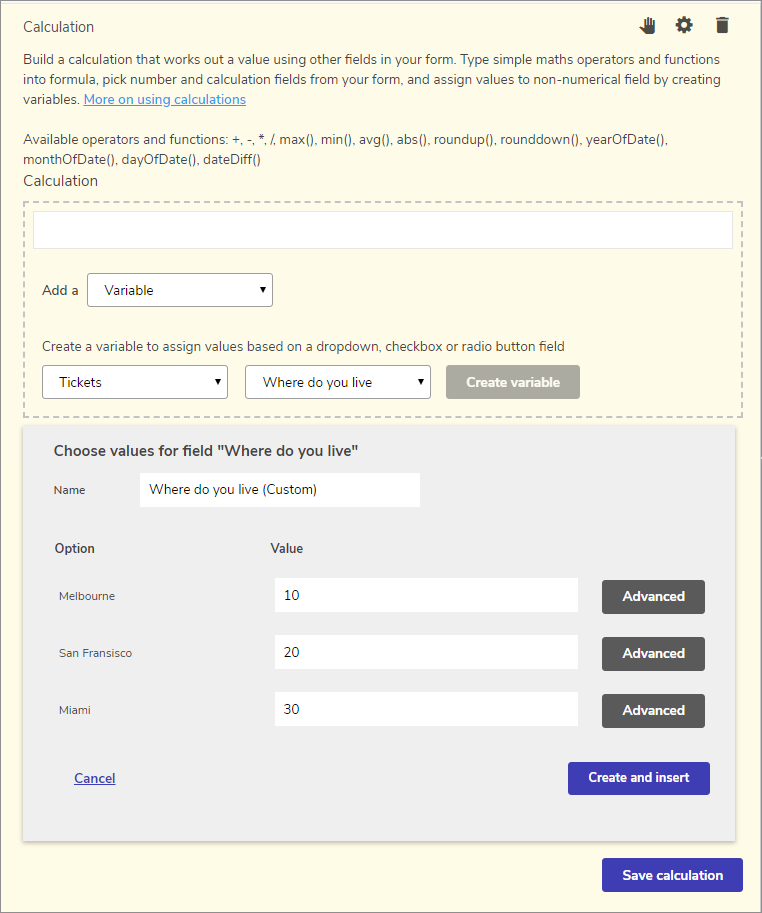5. Click Save calculation, then save your form.

We automatically display calculation fields as currency. If you don’t want this, open the field settings by choosing the gear icon and disable Display as currency.

Want to use your calculation in a payment field? Jump to Use a calculation in a payment field.

Still confused? Check out our calculation examples to get an idea of how to use them.

## Operators and functions

Use mathematical operators and functions to calculate the value you need.

### Operators

• - = Subtract

• * = Multiply

• / = Divide

To use an operation, type it in-between a number or another field, like this: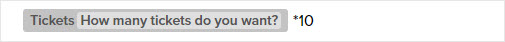In this example, the calculation will multiply the number of tickets by ten.

### Functions

• max() = Find the maximum value of multiple fields. Separate each field or number with a comma.
• min() = Find the minimum value of multiple fields. Separate each field or number with a comma.
• avg() = Find the average value of multiple fields. Separate each field or number with a comma.
• abs() = Find the absolute value of a field or number
• round() = Rounds a field or number to the nearest whole number, or within a decimal range (if you've specified one)
• roundup() = Round up a field or a number
• rounddown() = Round down a field or a number

When rounding numbers up, down, or to the nearest value, you can add a number after the field to specify how many decimal points to round to.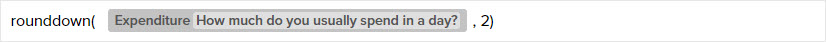• yearOfDate() = Find the year of a given date or date field
• monthOfDate() = Find the month of a given date or date field
• dayOfDate() = Find the day of a given date or date field
• dateDiff() = Find the difference between two given dates or date fields

When using yearOfDate()monthOfDate()dayOfDate(), or dateDiff(), you can add a date field, or type in a date yourself—just make sure you contain it within single quote marks. You can use any of these date formats:
yyyy-MM-dd, e.g. yearOfDate('2018-12-30')
d MMM yyyy, e.g. yearOfDate('30 Dec 2018')
d MMMM yyyy, e.g. yearOfDate('30 December 2018')

To use a function, put a field or number—or multiple fields or numbers—between the brackets like this: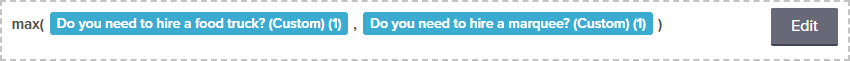In this example, the calculation will find the largest value of the two fields.

The calculation will still work fine if the last bracket of a function is on a second line.

## Make advanced calculations using variables

When you add a variable to a calculation you assign values to each option. If you want a more detailed way of setting an option’s value, click Advanced to open the rule builder. The rule builder lets you choose the earlier answers that will determine the option’s value. You can add as many rules per option as you like.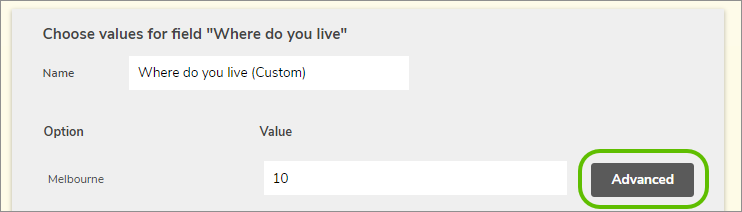To build a rule for the value, choose a section, a field, and a response to the field; then press Add. Set a value for the rule in the Value box.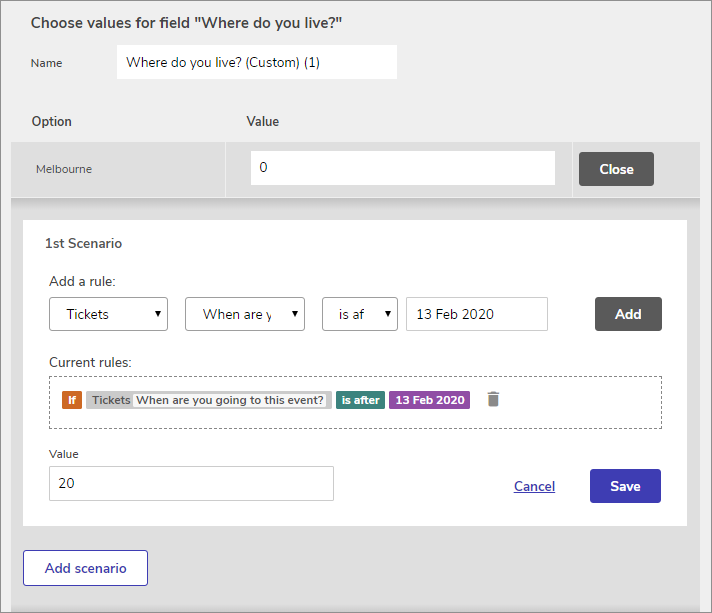In this example, if someone's answer to When are you going to this event? is after 13 February 2020, the value will be 20.

If you need an example to help you understand the rule builder better, check out our calculation examples.

## Use a calculation in a payment field

Once you’ve set up your calculation field, add a payment field to your form. Instead of typing a cost into the payment area, choose your new calculation field from the Use a calculation field value menu.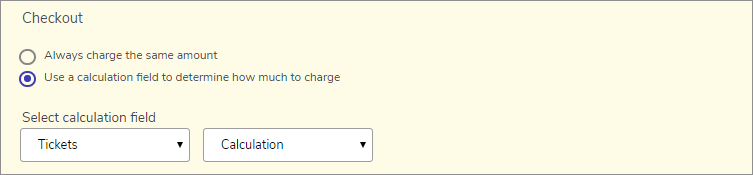Remember to save your form when you’re done.

When to hide your calculation field: Your calculation field will be automatically shown on your published form. If you're using it in a payment field, you'll see the same number twice, which you probably don't need. Visit Show or hide fields using display logic to find out how to hide your calculation field so it isn't shown to your form responders.

## Calculations applied to repeatable groups

When a calculation field references a field in a repeatable group the calculation will use the sum of every repetition of the referenced field. (Unless the calculation field is also inside the repeatable group).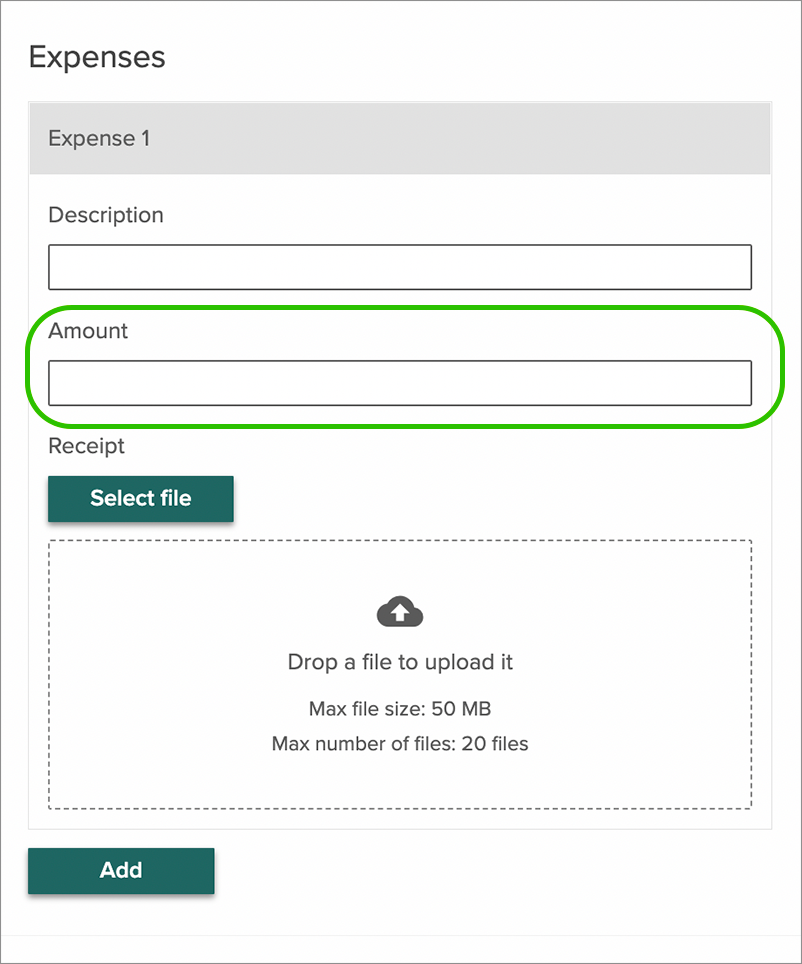In the example above, a repeatable group allows respondents to lodge itemized expenses, consisting of a description, an amount, and a receipt image for each expense.

Any calculation referencing the "amount" field will use the sum of every repetition of that field (however many the respondent chooses to submit), rather than one individual expense.

### Calculation functions and repeatable groups

Because some calculation functions (min, max, and avg) require at least two input values, they cannot currently be used to find the minimum, maximum, or average value of an individual field within a repeatable group. (As this is considered a single field in the calculation builder, even if there are multiple repetitions).

## What's next?

That’s everything you need to know about calculations. Check out our calculation examples for a deeper understanding, or visit Take payments through your form for more information on payments.

Tags: